# 1 Instalation

``````if (!require("BiocManager"))
install.packages("BiocManager")
BiocManager::install("glmSparseNet")``````

# 2 Required Packages

``````library(dplyr)
library(Matrix)
library(ggplot2)
library(forcats)
library(parallel)
library(reshape2)
library(survival)
library(VennDiagram)
library(loose.rock)
library(futile.logger)
library(TCGAutils)

library(glmSparseNet)

#
# Some general options for futile.logger the debugging package
.Last.value <- flog.layout(layout.format('[~l] ~m'))
.Last.value <- loose.rock::show.message(FALSE)

# Setting ggplot2 default theme as minimal
theme_set(ggplot2::theme_minimal())``````

# 3 Overview

This vignette uses the STRING database (https://string-db.org/) of protein-protein interactions as the network-based penalizer in generalized linear models using Breast invasive carcinoma sample dataset.

The degree vector is calculated manually to account for genes that are not present in the STRING database, as these will not have any interactions, i.e. edges.

Retrieve all interactions from STRING databse. We have included a helper function that retrieves the Homo sapiens known interactions.

For this vignette, we use a cached version of all interaction with `score_threshold = 700`

Note: Text-based interactions are excluded from the network.

``````# Not evaluated in vignette as it takes too long to download and process
all.interactions.700 <- stringDBhomoSapiens(score_threshold = 700)
string.network       <- buildStringNetwork(all.interactions.700,
use.names = 'external')``````

# 4 Build network matrix

Build a sparse matrix object that contains the network.

``````string.network.undirected <- string.network + Matrix::t(string.network)
string.network.undirected <- (string.network.undirected != 0) * 1``````

# 5 Network Statistics

## 5.1 Graph information

``````## [INFO] Directed graph (score_threshold = 700)
## [INFO]   *       total edges: 225330
## [INFO]   *    unique protein: 11033
## [INFO]   * edges per protein: 20.423276
## [INFO]
## [INFO] Undirected graph (score_threshold = 700)
## [INFO]   *       total edges: 225209
## [INFO]   *    unique protein: 11033
## [INFO]   * edges per protein: 20.412309``````

## 5.2 Summary of degree (indegree + outdegree)

``````## [INFO] Summary of degree:
##
##    Min. 1st Qu.  Median    Mean 3rd Qu.    Max.
##    1.00    3.00   13.00   40.83   43.00 4175.00``````

## 5.3 Histogram of degree (up until 99.999% quantile)

``````qplot(degree.network[degree.network <= quantile(degree.network,
probs = .99999)],
geom = 'histogram', fill = my.colors(2), bins = 100) +
theme(legend.position = 'none') + xlab('Degree (up until 99.999% quantile)')``````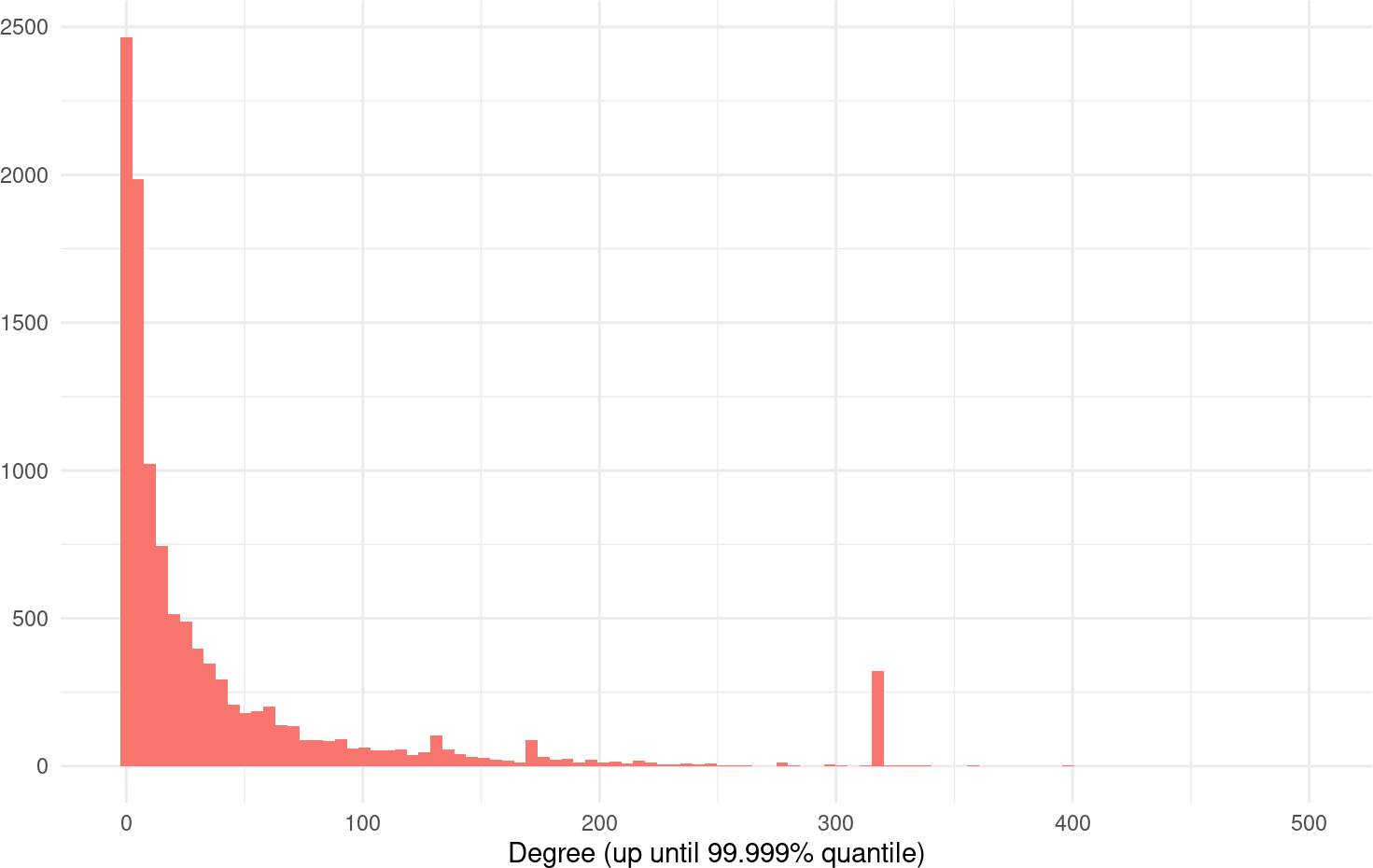# 6`glmSparseNet`

``````brca <- curatedTCGAData(diseaseCode = "BRCA", assays = "RNASeq2GeneNorm",
version = "1.1.38", dry.run = FALSE)``````

Build the survival data from the clinical columns.

• Selects only primary solid tumour samples
• Merge survival times for patients, which have different columns in case they are alive or dead.
• Build two matrix objects that fit the data `xdata` and `ydata`
``````# keep only solid tumour (code: 01)
brca.primary.solid.tumor <- TCGAutils::TCGAsplitAssays(brca, '01')
xdata.raw                <- t(assay(brca.primary.solid.tumor[]))

# Get survival information
ydata.raw <- colData(brca.primary.solid.tumor) %>% as.data.frame %>%
# Convert days to integer
dplyr::mutate(
Days.to.date.of.Death = as.integer(Days.to.date.of.Death),
Days.to.Last.Contact  = as.integer(Days.to.Date.of.Last.Contact)
) %>%
# Find max time between all days (ignoring missings)
dplyr::rowwise() %>%
dplyr::mutate(
time = max(days_to_last_followup, Days.to.date.of.Death,
Days.to.Last.Contact, days_to_death, na.rm = TRUE)
) %>%
# Keep only survival variables and codes
dplyr::select(patientID, status = vital_status, time) %>%
# Discard individuals with survival time less or equal to 0
dplyr::filter(!is.na(time) & time > 0) %>%
as.data.frame()

# Set index as the patientID
rownames(ydata.raw) <- ydata.raw\$patientID

# keep only features that are in degree.network and have standard deviation > 0
valid.features <- colnames(xdata.raw)[colnames(xdata.raw) %in%
names(degree.network[degree.network > 0])]
xdata.raw      <- xdata.raw[TCGAbarcode(rownames(xdata.raw)) %in%
rownames(ydata.raw), valid.features]
xdata.raw      <- scale(xdata.raw)

# Order ydata the same as assay
ydata.raw    <- ydata.raw[TCGAbarcode(rownames(xdata.raw)), ]

# Using only a subset of genes previously selected to keep this short example.
set.seed(params\$seed)
small.subset <- c('AAK1', 'ADRB1', 'AK7', 'ALK', 'APOBEC3F', 'ARID1B', 'BAMBI',
'BRAF', 'BTG1', 'CACNG8', 'CASP12', 'CD5',  'CDA', 'CEP72',
'CPD', 'CSF2RB', 'CSN3', 'DCT', 'DLG3',  'DLL3', 'DPP4',
'DSG1', 'EDA2R', 'ERP27', 'EXD1', 'GABBR2',  'GADD45A',
'GBP1', 'HTR1F', 'IFNK', 'IRF2', 'IYD', 'KCNJ11',  'KRTAP5-6',
'MAFA', 'MAGEB4', 'MAP2K6', 'MCTS1', 'MMP15', 'MMP9',
'NFKBIA', 'NLRC4', 'NT5C1A', 'OPN4', 'OR13C5', 'OR13C8',
'OR2T6', 'OR4K2', 'OR52E6', 'OR5D14', 'OR5H1', 'OR6C4',
'OR7A17', 'OR8J3',  'OSBPL1A', 'PAK6', 'PDE11A', 'PELO',
'PGK1', 'PIK3CB', 'PMAIP1',  'POLR2B', 'POP1', 'PPFIA3',
'PSME1', 'PSME2', 'PTEN', 'PTGES3',  'QARS', 'RABGAP1',
'RBM3', 'RFC3', 'RGPD8', 'RPGRIP1L', 'SAV1',  'SDC1', 'SDC3',
'SEC16B', 'SFPQ', 'SFRP5', 'SIPA1L1', 'SLC2A14', 'SLC6A9',
'SPATA5L1', 'SPINT1', 'STAR', 'STXBP5', 'SUN3', 'TACC2',
'TACR1', 'TAGLN2', 'THPO', 'TNIP1', 'TP53', 'TRMT2B', 'TUBB1',
'VDAC1', 'VSIG8', 'WNT3A', 'WWOX', 'XRCC4', 'YME1L1',
'ZBTB11',  'ZSCAN21') %>%
sample(size  = 50) %>% sort

# make sure we have 100 genes
small.subset <- c(small.subset, sample(colnames(xdata.raw), 51)) %>%
unique %>%
sort

xdata <- xdata.raw[, small.subset[small.subset %in% colnames(xdata.raw)]]
ydata <- ydata.raw %>%
dplyr::select(time, status) %>%
dplyr::filter(!is.na(time) | time < 0)``````
• Build dataset that overlaps with STRING data
``````#
# Add degree 0 to genes not in STRING network

my.degree <- degree.network[small.subset]
my.string <- string.network.binary[small.subset, small.subset]``````

Degree distribution for sample set of gene features (in xdata).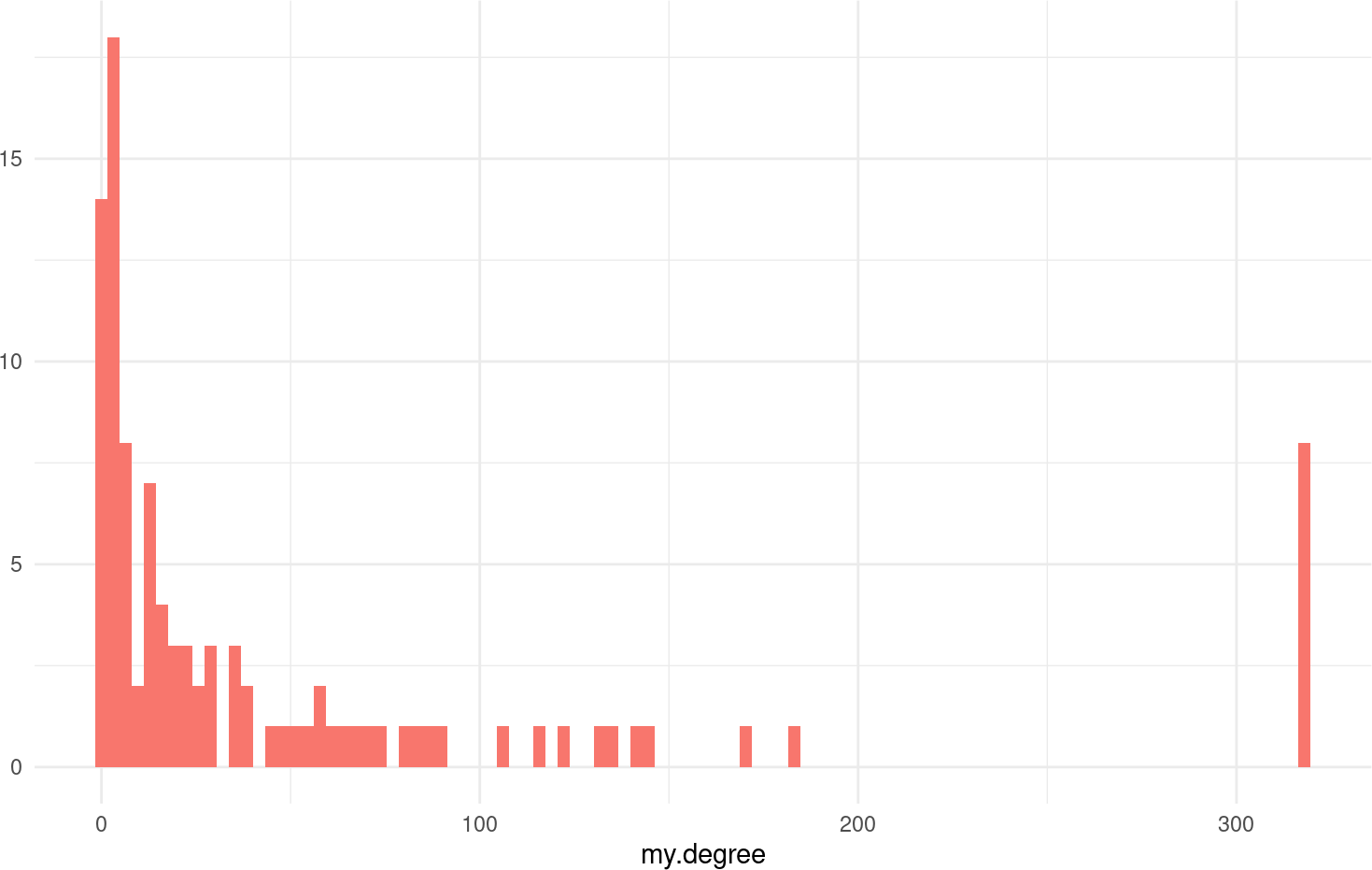## 6.1 Select balanced folds for cross-validation

``````set.seed(params\$seed)
foldid <- balanced.cv.folds(!!ydata\$status)\$output``````

## 6.2 glmHub model

Penalizes using the Hub heuristics, see `hubHeuristic` function definition for more details.

``````cv.hub <- cv.glmHub(xdata,
Surv(ydata\$time, ydata\$status),
family  = 'cox',
foldid  = foldid,
network = my.string,
network.options = networkOptions(min.degree = 0.2))``````

Kaplan-Meier estimator separating individuals by low and high risk (based on model’s coefficients)

``````## \$pvalue
##  0
##
## \$plot``````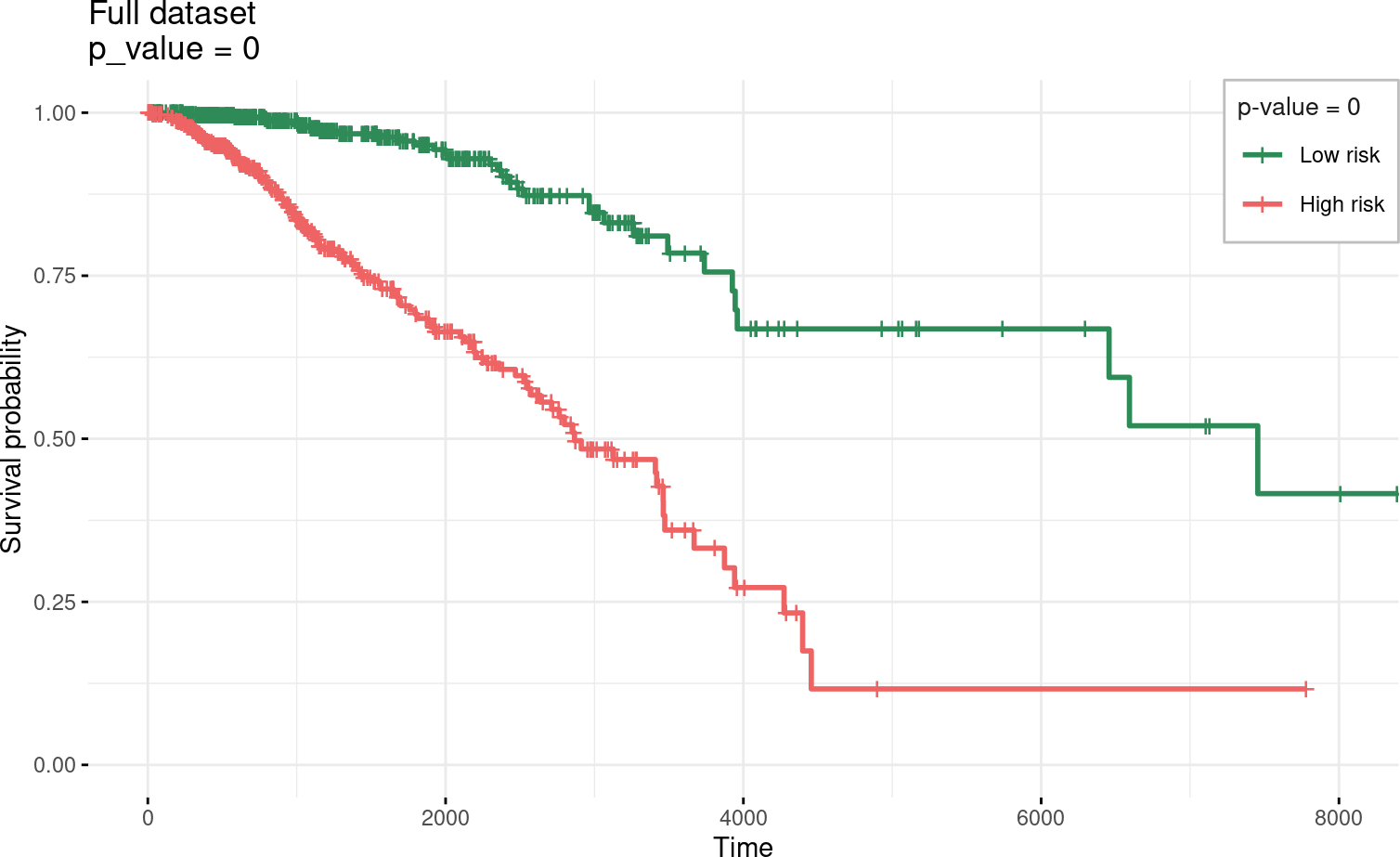``````##
## \$km
## Call: survfit(formula = survival::Surv(time, status) ~ group, data = prognostic.index.df)
##
##             n events median 0.95LCL 0.95UCL
## Low risk  540     34   7455    6456      NA
## High risk 540    118   2866    2573    3472``````

## 6.3 glmOrphan model

Penalizes using the Orphan heuristics, see `orphanHeuristic` function definition for more details.

``````cv.orphan <- cv.glmOrphan(xdata,
Surv(ydata\$time, ydata\$status),
family  = 'cox',
foldid  = foldid,
network = my.string,
network.options = networkOptions(min.degree = 0.2))``````

Kaplan-Meier estimator separating individuals by low and high risk (based on model’s coefficients)

``````## \$pvalue
##  0
##
## \$plot``````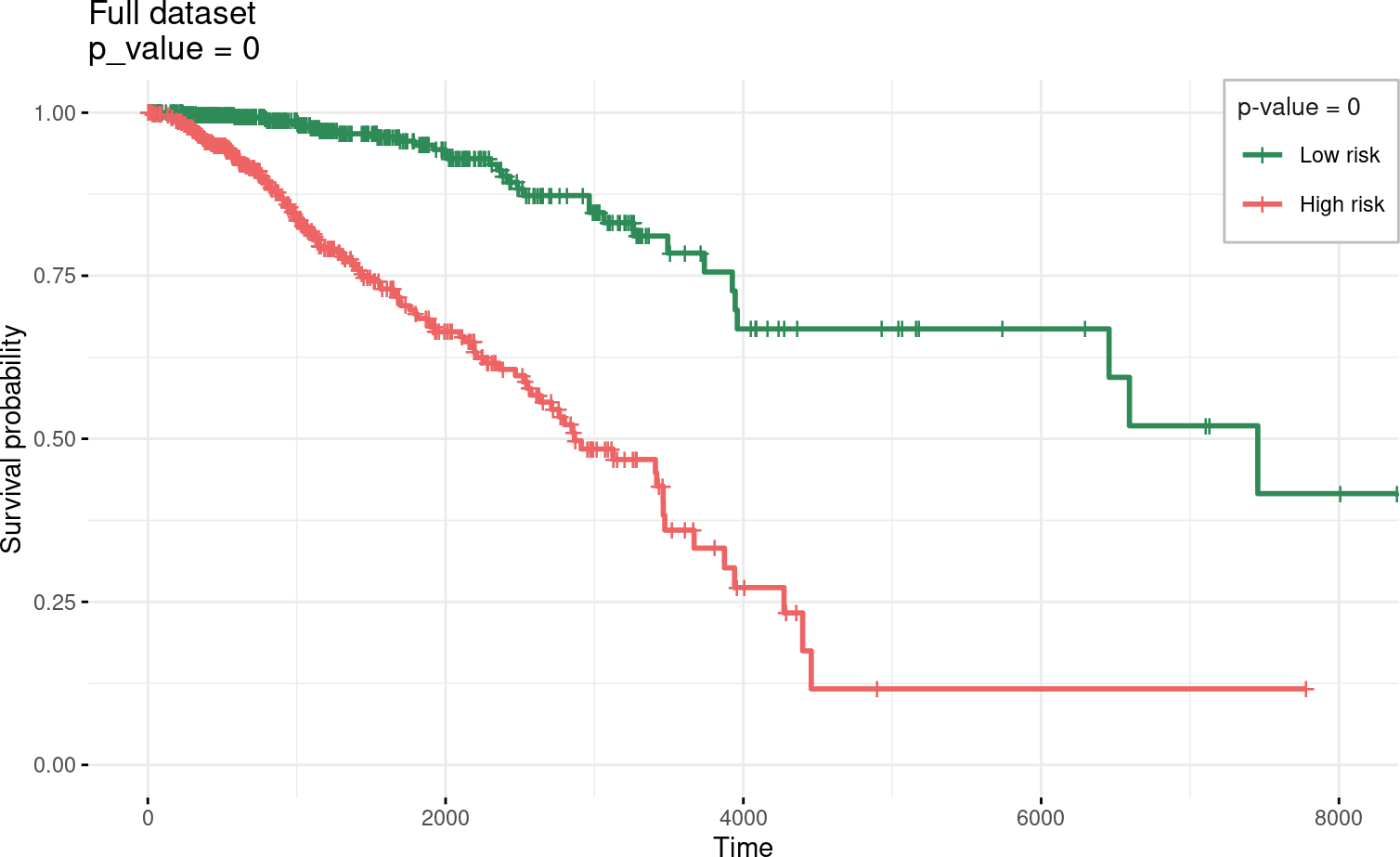``````##
## \$km
## Call: survfit(formula = survival::Surv(time, status) ~ group, data = prognostic.index.df)
##
##             n events median 0.95LCL 0.95UCL
## Low risk  540     34   7455    6456      NA
## High risk 540    118   2866    2573    3472``````

## 6.4 Elastic Net model (without network-penalization)

Uses regular glmnet model as simple baseline

``````cv.glm <- cv.glmnet(xdata,
Surv(ydata\$time, ydata\$status),
family = 'cox',
foldid = foldid)``````

Kaplan-Meier estimator separating individuals by low and high risk (based on model’s coefficients)

``````## \$pvalue
##  0
##
## \$plot``````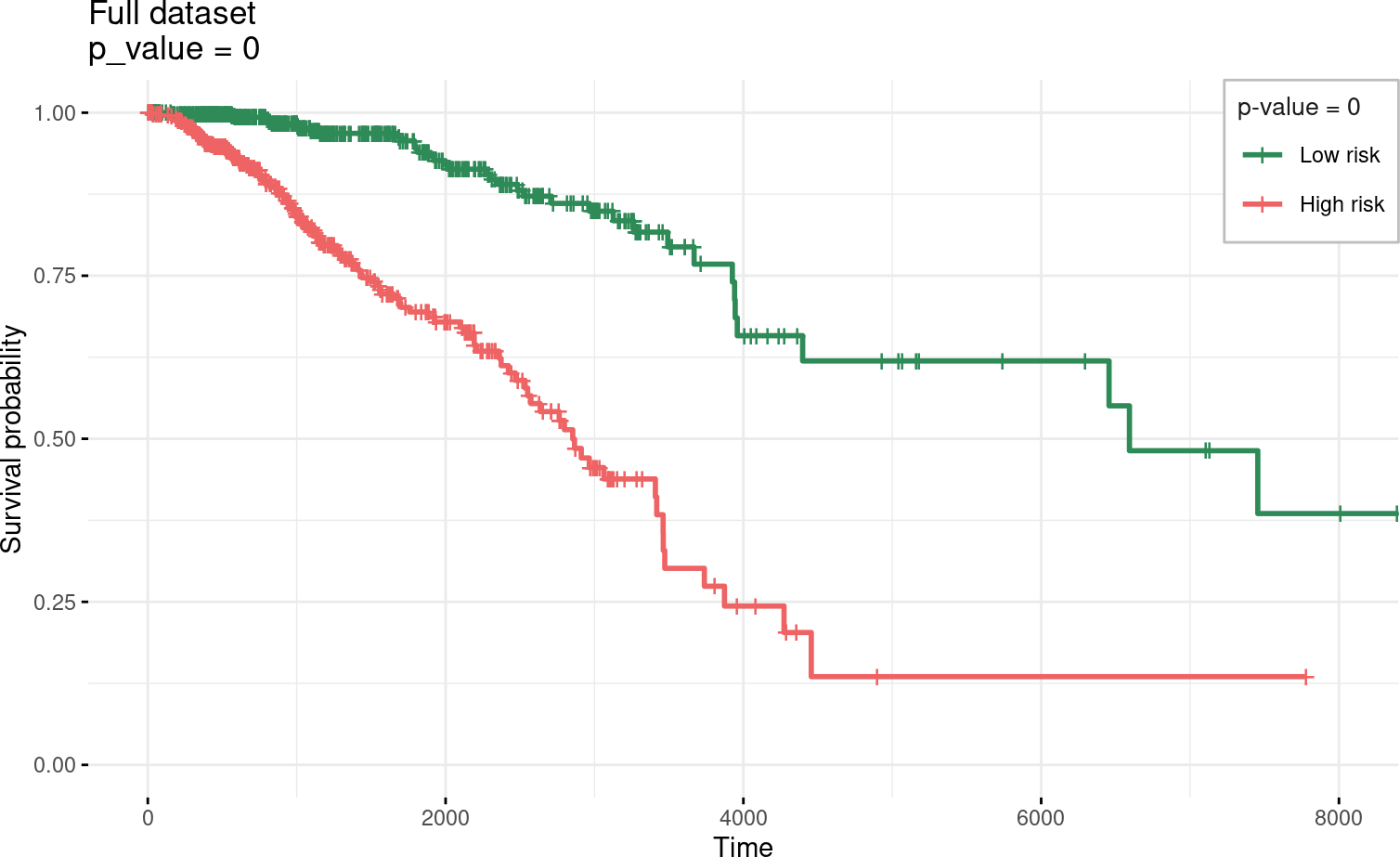``````##
## \$km
## Call: survfit(formula = survival::Surv(time, status) ~ group, data = prognostic.index.df)
##
##             n events median 0.95LCL 0.95UCL
## Low risk  540     38   6593    4398      NA
## High risk 540    114   2854    2551    3461``````

## 6.5 Selected genes

Venn diagram of overlapping genes.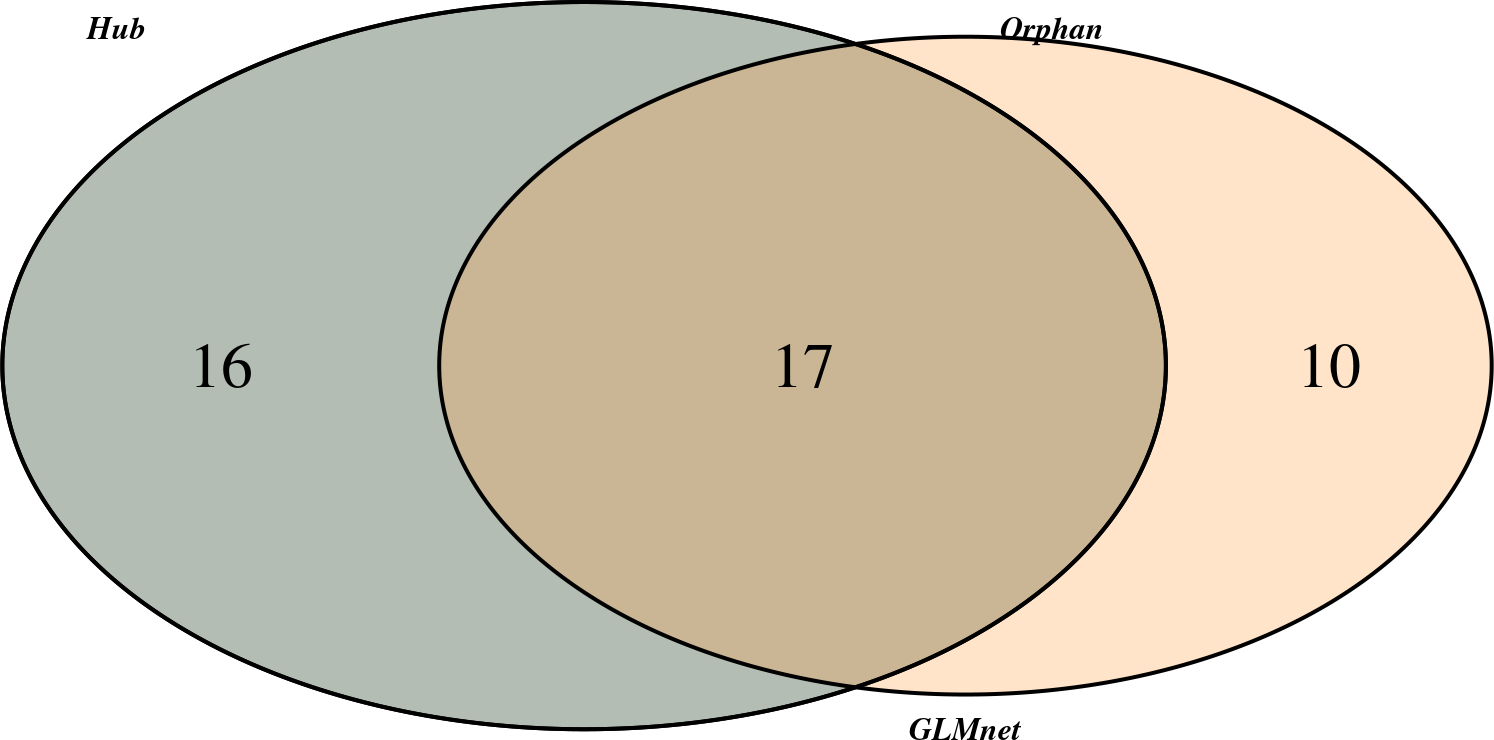Descriptive table showing which genes are selected in each model

We can observe, that elastic net without network-based penalization selects the best model with 40% more genes than glmOrphan and glmHub, without loosing accuracy.

note: size of circles represent the degree of that gene in network.